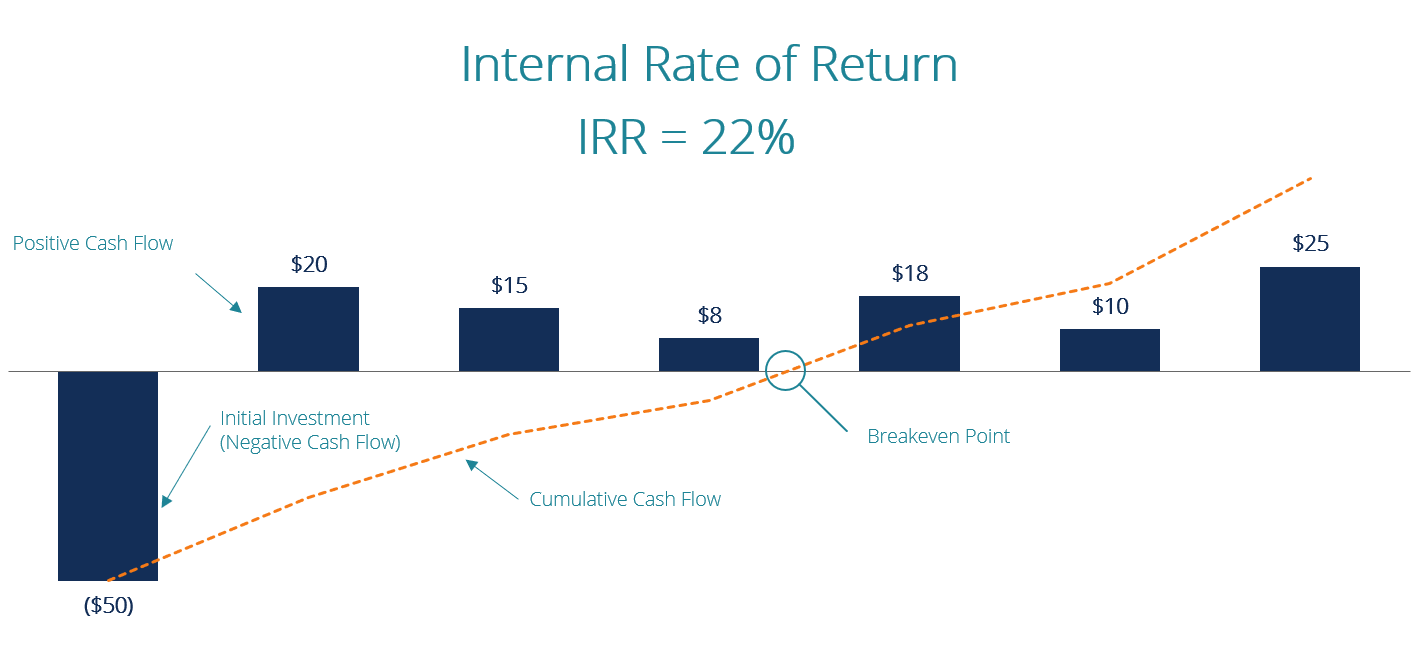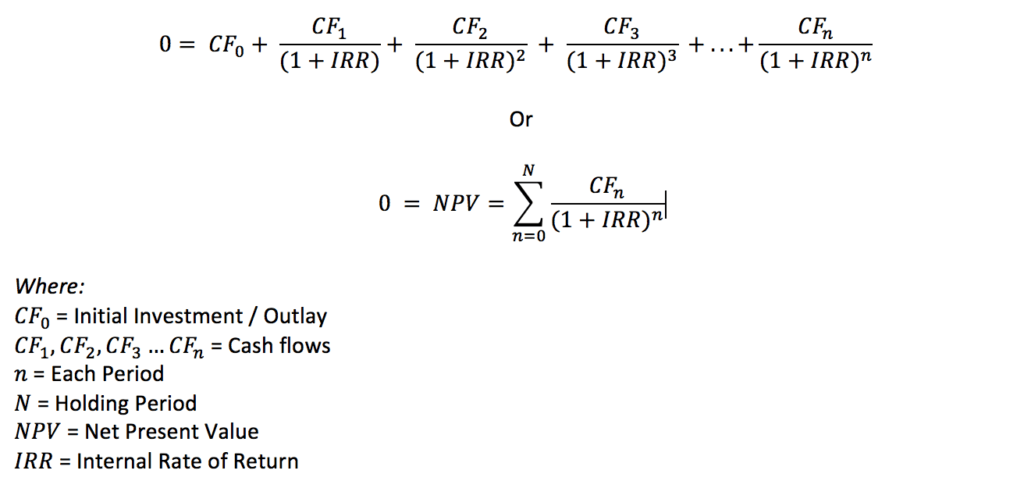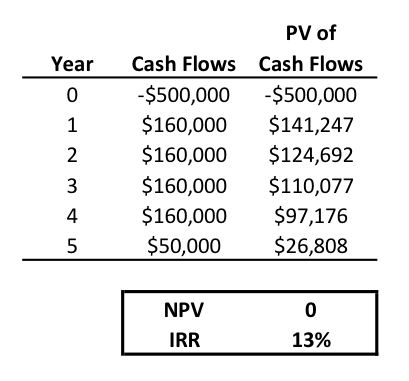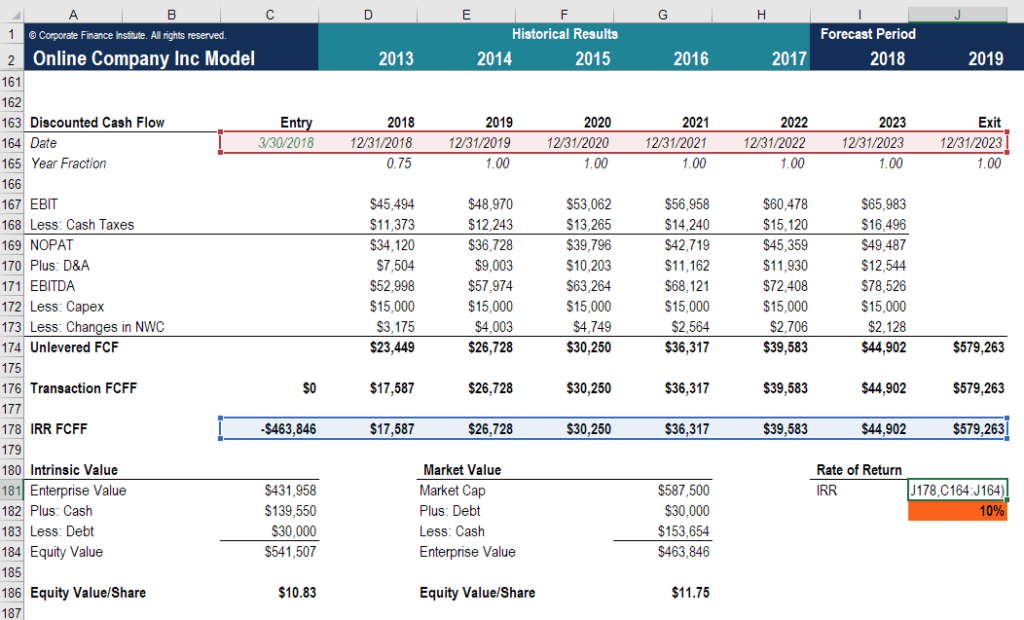# Internal Rate of Return (IRR)

An Analyst's Guide to IRR

## What is Internal Rate of Return (IRR)?

The Internal Rate of Return (IRR) is the discount rate that makes the net present value (NPV) of a project zero. In other words, it is the expected compound annual rate of return that will be earned on a project or investment. In the example below, an initial investment of \$50 has a 22% IRR. That is economically equal to earning a 22% compound annual growth rate.When calculating IRR, expected cash flows for a project or investment are given and the NPV equals zero. Put another way, the initial cash investment for the beginning period will be equal to the present value of the future cash flows of that investment. (Cost paid = present value of future cash flows, and hence, the net present value = 0).

Once the internal rate of return is determined, it is typically compared to a company’s hurdle rate or cost of capital. If the IRR is greater than or equal to the cost of capital, the company would accept the project as a good investment. (That is, of course, assuming this is the sole basis for the decision. In reality there are many other quantitative and qualitative factors that are considered in an investment decision.) If the IRR is lower than the hurdle rate, then it would be rejected.

### What is the IRR Formula?

The IRR formula is as follows:Calculating the internal rate of return can be done in three ways:

1. Using the IRR or XIRR function in Excel or other spreadsheet programs (see example below)
2. Using a financial calculator
3. Using an iterative process where the analyst tries different discount rates until the NPV equals to zero (Goal Seek in Excel can be used to do this)

### Example of Calculating IRR

Here is an example of how to calculate the Internal Rate of Return.

A company is deciding whether to purchase new equipment that costs \$500,000. Management estimates the life of the new asset to be four years and expects it to generate an additional \$160,000 of annual profits. In the fifth year, the company plans to sell the equipment for its salvage value of \$50,000.

Meanwhile, another similar investment option can generate a 10% return. This is higher than the company’s current hurdle rate of 8%. The goal is to make sure the company is making better use of its cash.

To make a decision, the IRR for investing in the new equipment is calculated below.

Excel was used to calculate the IRR of 13%, using the function, =IRR(). From a financial standpoint, the company should make the purchase, because the IRR is both greater than the hurdle rate and the IRR for the alternative investment.### What is Internal Rate of Return Used For?

Companies take on various projects to increase their revenues or cut down costs. A great new business idea may require, for example, investing in the development of a new product.

In capital budgeting, senior leaders like to know the reasonably projected returns on such investments. The internal rate of return is one method that allows them to compare and rank projects based on their projected yield. The investment with the highest internal rate of return is usually preferred.

Internal Rate of Return is widely used in analyzing investments for private equity and venture capital, which involves multiple cash investments over the life of a business and a cash flow at the end through an IPO or sale of the business.

Thorough investment analysis requires an analyst to examine both the net present value (NPV) and the internal rate of return, along with other indicators, such as the payback period, in order to select the right investment.  Since it’s possible for a very small investment to have a very high rate of return, investors and managers sometimes choose a lower percentage return but higher absolute dollar value opportunity. Also, it’s important to have a good understanding of your own risk tolerance, or a company’s investment needs, risk aversion, and other available options.

### Video Explanation of Internal Rate of Return (IRR)

Below is a short video explanation with an example of how to use the XIRR function in Excel to calculate the internal rate of return of an investment. The demonstration shows how the IRR is equal to the compound annual growth rate (CAGR).

### What IRR Really Means (Another Example)

Let’s look at an example of a financial model in Excel to see what the internal rate of return number really means.

If an investor paid \$463,846 (which is the negative cash flow shown in cell C178) for a series of positive cash flows as shown in cells D178 to J178, the IRR they would receive is 10%. This means the net present value of all these cash flows (including the negative outflow) is zero and that only the 10% rate of return is earned.

If the investors paid less than \$463,846 for all same additional cash flows, then their IRR would be higher than 10%. Conversely, if they paid more than \$463,846, then their IRR would be lower than 10%.The above screenshot is from CFI’s M&A Modeling Course.

Unlike net present value, the internal rate of return doesn’t give you the return on initial investment in terms of real dollars. For example, knowing an IRR of 30% alone doesn’t tell you if it’s 30% of \$10,000 or 30% of \$1,000,000.

Using IRR exclusively can lead you to make poor investment decisions, especially if comparing two projects with different durations.

Let’s say a company’s hurdle rate is 12%, and one-year project A has an IRR of 25%, whereas five-year project B has an IRR of 15%. If the decision is solely based on IRR, this would lead to unwisely choosing project A over B.

Another very important point about the internal rate of return is that it assumes all positive cash flows of a project will be reinvested at the same rate as the project, instead of the company’s cost of capital. Therefore, internal rate of return may not accurately reflect the profitability and cost of a project.

A smart financial analyst will alternatively use the modified internal rate of return (MIRR) to arrive at a more accurate measure.

• XIRR vs IRR
• Weighted Average Cost of Capital (WACC)
• Hurdle Rate

### Financial Analyst Training

Get world-class financial training with CFI’s online certified financial analyst training program!

Gain the confidence you need to move up the ladder in a high powered corporate finance career path.

Learn financial modeling and valuation in Excel the easy way, with step-by-step training.